Test: Pulse Amplitude Modulation (PAM)

# Test: Pulse Amplitude Modulation (PAM)

Test Description

## 10 Questions MCQ Test GATE ECE (Electronics) 2023 Mock Test Series | Test: Pulse Amplitude Modulation (PAM)

Test: Pulse Amplitude Modulation (PAM) for Electronics and Communication Engineering (ECE) 2023 is part of GATE ECE (Electronics) 2023 Mock Test Series preparation. The Test: Pulse Amplitude Modulation (PAM) questions and answers have been prepared according to the Electronics and Communication Engineering (ECE) exam syllabus.The Test: Pulse Amplitude Modulation (PAM) MCQs are made for Electronics and Communication Engineering (ECE) 2023 Exam. Find important definitions, questions, notes, meanings, examples, exercises, MCQs and online tests for Test: Pulse Amplitude Modulation (PAM) below.
Solutions of Test: Pulse Amplitude Modulation (PAM) questions in English are available as part of our GATE ECE (Electronics) 2023 Mock Test Series for Electronics and Communication Engineering (ECE) & Test: Pulse Amplitude Modulation (PAM) solutions in Hindi for GATE ECE (Electronics) 2023 Mock Test Series course. Download more important topics, notes, lectures and mock test series for Electronics and Communication Engineering (ECE) Exam by signing up for free. Attempt Test: Pulse Amplitude Modulation (PAM) | 10 questions in 30 minutes | Mock test for Electronics and Communication Engineering (ECE) preparation | Free important questions MCQ to study GATE ECE (Electronics) 2023 Mock Test Series for Electronics and Communication Engineering (ECE) Exam | Download free PDF with solutions
 1 Crore+ students have signed up on EduRev. Have you?
Test: Pulse Amplitude Modulation (PAM) - Question 1

### Which of the following systems is analog?

Detailed Solution for Test: Pulse Amplitude Modulation (PAM) - Question 1
• Analog modulation is a process in which analog low-frequency baseband signals (like an audio or TV signal) are transmitted over a larger distance without getting faded away, by superimposing over a higher frequency carrier signal such as a radio frequency band.
• Different modulation techniques are explained with the help of the following block diagram: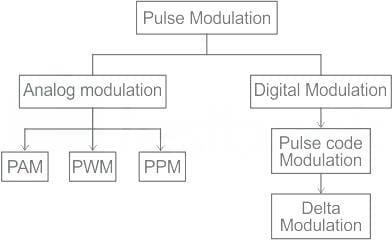• If the amplitude of a pulse or duration of a pulse is varied according to the instantaneous values of the baseband modulating signal, then such a technique is called as Pulse Amplitude Modulation (PAM).
Test: Pulse Amplitude Modulation (PAM) - Question 2

### A PAM signal can be detected by using

Detailed Solution for Test: Pulse Amplitude Modulation (PAM) - Question 2

A PAM signal is demodulated using an integrator. Signals having same width but different amplitudes produce different values at the output of an integrator.

Test: Pulse Amplitude Modulation (PAM) - Question 3

### Which filter is used to detect the PAM signal?

Detailed Solution for Test: Pulse Amplitude Modulation (PAM) - Question 3

PAM (Pulse Amplitude Modulation):

• It is a technique in which the amplitude of each pulse is controlled by the instantaneous amplitude of the modulation signal.
•  It is a modulation system in which the signal is sampled at regular intervals and each sample is made proportional to the amplitude of the signal at the instant of sampling.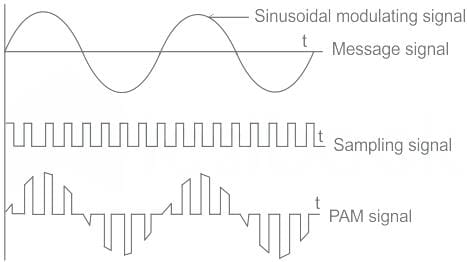• The demodulation of the PAM signals is done by using a Low-pass filter as shown: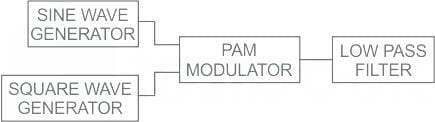Test: Pulse Amplitude Modulation (PAM) - Question 4

A speech signal is sampled a rate of 20% above the Nyquist rate. The signal has a bandwidth of 10 kHz. The sample is quantized into 1024 levels and then transmitted through 8-level PAM over an AWGN baseband channel. The bandwidth required for transmission is ______

Detailed Solution for Test: Pulse Amplitude Modulation (PAM) - Question 4

Concept: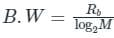Where,
Rb = nfs
n = no. of bits
M = no. of levels of PAM
Calculation:
fm = 10 kHz
Nyquist sampling rate = 2f­m = 20 kHz.
The signal is sampled at 20% above Nyquist rate:
∴ fs = (1.2 × 20) = 24 kHz.
No. of Quantization levels
= 1024
No. of bits required = log2 (1024)
= log2 210 = 10 bits.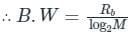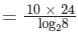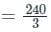= 80 kHz.

Test: Pulse Amplitude Modulation (PAM) - Question 5

Which of the following Pulse time Modulation does not exist in practice?

Detailed Solution for Test: Pulse Amplitude Modulation (PAM) - Question 5

PFM does not exist in practice

PWM

The amplitude and position of pulses are constant in modulated signal (PWM), but the width (or duration) of pulses varies proportionally with the amplitude of an analog useful signal.
The carrier signal is produced by a clock.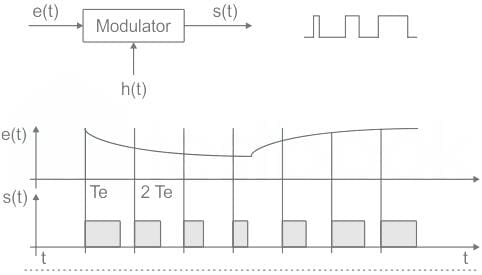PPM
The amplitude and width of pulses are constant in a modulated signal (PPM), but the direction of pulses varies proportionally with the amplitude of analogical useful signal.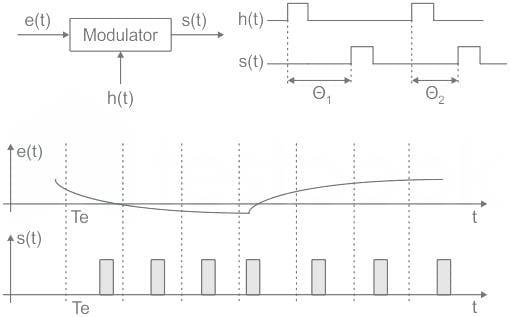PAM
The width and location of pulses in a modulated signal (PAM) are constant, while the amplitude of pulses varies proportionally with the amplitude of an analogical useful signal.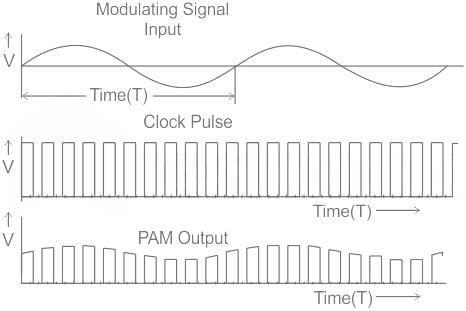Test: Pulse Amplitude Modulation (PAM) - Question 6

Study the given input signal and match the columns.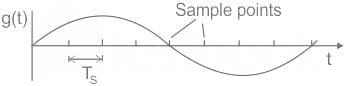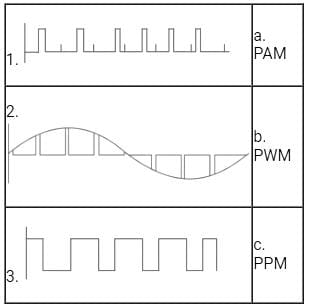Detailed Solution for Test: Pulse Amplitude Modulation (PAM) - Question 6

PFM does not exist in practice

PWM

The amplitude and position of pulses are constant in modulated signal (PWM), but the width (or duration) of pulses varies proportionally with the amplitude of an analog useful signal.
The carrier signal is produced by a clock.PPM
The amplitude and width of pulses are constant in a modulated signal (PPM), but the direction of pulses varies proportionally with the amplitude of analogical useful signal.PAM
The width and location of pulses in a modulated signal (PAM) are constant, while the amplitude of pulses varies proportionally with the amplitude of an analogical useful signal.Hence from the above option (d) is correct

Test: Pulse Amplitude Modulation (PAM) - Question 7

A PAM source generates four symbols 3 V, 1 V, -1 V and -3 V with probability of p(3) = p(-3) = 0.2 and p(1) = p(-1) = 0.3 respectively. The variance for the source will be

Detailed Solution for Test: Pulse Amplitude Modulation (PAM) - Question 7

Concept:
For discrete random variable x:
Mean = E[x] = ∑I xi p (xi)
Mean square value (MSQ) = E[x2] = ∑I xi2 p (xi)
Variance (σ2) = E[x2] – {E(x)}2
Standard deviation (σ) =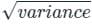Calculation:
Given data: for the given PAM source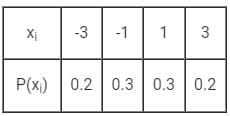Mean = E[x] = ∑i xi p (xi)
= (-3)(0.2) + (-1)(0.3) + (1)(0.3) + (3)(0.2)
= 0
MSQ = E[x2] = ∑I xi2 p (xi)
= (-3)2(0.2) + (-1)2(0.3) + (1)2(0.3) + (3)2(0.2) V
= 4.2 V
Variance = E[x2] – {E[x]}2
= 4.2 – 0
= 4.2 V

Test: Pulse Amplitude Modulation (PAM) - Question 8

Consider a baseband binary PAM receiver shown below. The additive channel noise n(t) is white with power spectral density SN(f) = N0/2 = 10-20 W/Hz. The low-pass filter is ideal with unity gain and cutoff frequency 1 MHz. Let Yt, represent the random variable y(t1)
Yt = Ns if transmitted bit bk = 0
YK = a + Nk if transmitted bit bk = 1
where Nk represents the noise sample value. The noise sample has a probability density function, PN (n) = 0.5αe (This has mean zero and variance 2/α2), Assume transmitted bits lo be equiprobable and threshold z is set to a/2 = 10-6 V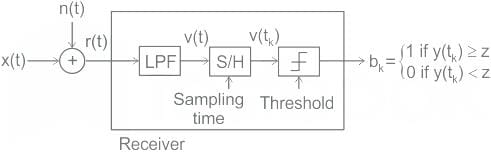‘The probability of bit error is

Detailed Solution for Test: Pulse Amplitude Modulation (PAM) - Question 8

Concept:
Consider the block diagram as shown below to represent the noise which is treated as error: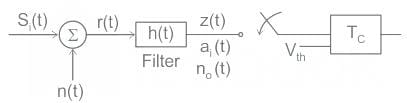r(t) = Si(t) + n(t)
The output of the filter is:
z(t) = ai(t) + n0(t)
ai(t): Signal component
n0(t): Noise component
The probability of error is represented in terms of the Q function: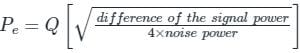Pe = Q[x]
When the pdf's are not having an equal width then the probability of error will not be the same for both symbols. In this case, the Bit error rate is calculated.
BER = P(1) × Pe1 + P(0) × Pe0
P(1): Probability when 1 is transmitted
P(0): Probability when 0 transmitted
Pe0: Probability of error when 0 transmitted
Pe1: Probability of error when 1 transmitted.
Calculation:
The system is defined as shown: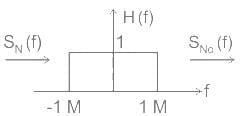The given mean is 0 and variance is 2/α2
V[X] = E[X2] - (E[X])2
2/α2 = E[X2]
The area under the curve is: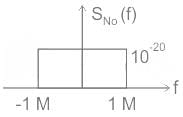E[X2] = 10-20 × 2 × 106
2/α2 = 10-14 × 2
α2 = 1014
α = 107
Given Yt, represent the random variable y(t1)
Yt = Ns if transmitted bit bk = 0
YK = a + Nk if transmitted bit bk = 1 and threshold z is set to a/2 = 10-6 V
P(0) = P(1) = 1/2
Pe = P(1) × Pe1 + P(0) × Pe0
Pe1 calculation
We know that when the output signal is less than the threshold it is considered as 0 and there is an error when 1 is transmitted.
Yk < z ⇒ error
a + Nk < 10-6
Nk < -10-6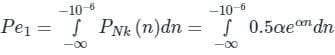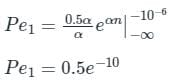Pe0 calculation
We know that when the output signal is more than the threshold it is considered as 1 and there is an error when 0 is transmitted.
Yk > z ⇒ error
Ns > 10-6
Ns > -10-6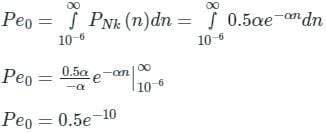The probability of error is: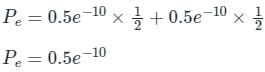Test: Pulse Amplitude Modulation (PAM) - Question 9

Consider a baseband binary PAM receiver shown below. The additive channel noise n(t) is white with power spectral density SN(f) = N0/2 = 10-20 W/Hz. The low-pass filter is ideal with unity gain and cutoff frequency 1 MHz. Let Yt, represent the random variable y(t1)
Yt = Ns if transmitted bit bk = 0
YK = a + Nk if transmitted bit bk = 1
where Nk represents the noise sample value. The noise sample has a probability density function, PN (n) = 0.5αe (This has mean zero and variance 2/α2), Assume transmitted bits lo be equiprobable and threshold z is set to a/2 = 10-6 VQ. The value of the parameter α (in V-1) is

Detailed Solution for Test: Pulse Amplitude Modulation (PAM) - Question 9

Concept:
The statistical averages Mean and Variance are called as 'first order moment' and 'second-order moment' respectively.
Variance represents the total power of the random signal.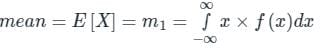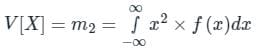Total power can be determined as the area of the curve.
When the input is passed through a system the output is shown by: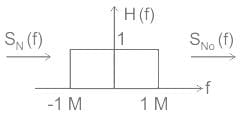SN(f): Input noise PSD
SN0(f): Output noise PSD
H(f): system response
Calculation:
The system is defined as shown: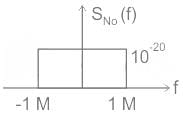The given mean is 0 and variance is 2/α2
V[X] = E[X2] - (E[X])2
2/α2 = E[X2]
The area under the curve is:
E[X2] = 10-20 × 2 × 106
2/α2 = 10-14 × 2
α2 = 1014
α = 107

Test: Pulse Amplitude Modulation (PAM) - Question 10

Which of the following is NOT an advantage of Pulse Duration Modulation (PDM) recording?

Detailed Solution for Test: Pulse Amplitude Modulation (PAM) - Question 10

Concept:
Pulse Width Modulation (PWM):
As mentioned PWM is short form of Pulse Width Modulation in which width of pulse is proportional to amplitude of the modulating signal.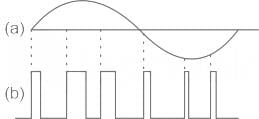Following are the benefits or advantages of PWM:

• Noise interference is less or minimum.
• The system is moderate in complexity to implement.
• It has moderate power efficiency among all three types.
• It supports higher power handling capability.
• It has high accuracy due to the fact that it can be self-calibrated.
• It has high S/N ratio.
• It has the ability to simultaneously record information from a large number of channels.

Following are the disadvantages of PWM:

• Instantaneous power of transmitter varies.
• The system requires semiconductor devices with low turn-ON and turn-OFF times. Hence they are very expensive.
• High switching losses due to higher PWM frequency.

From the given statements, 'It has a less complex electronic circuitry and therefore the reliability of such system is high' is NOT an advantage of Pulse Duration Modulation (PDM) recording.

## GATE ECE (Electronics) 2023 Mock Test Series

21 docs|263 tests
Information about Test: Pulse Amplitude Modulation (PAM) Page
In this test you can find the Exam questions for Test: Pulse Amplitude Modulation (PAM) solved & explained in the simplest way possible. Besides giving Questions and answers for Test: Pulse Amplitude Modulation (PAM), EduRev gives you an ample number of Online tests for practice

## GATE ECE (Electronics) 2023 Mock Test Series

21 docs|263 tests(Scan QR code)